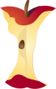The Area of RectanglesPrintable Worksheets And Lessons

• Step-by-step Lesson- Understand how to use standard units of a rectangle to calculate area.

• Guided Lesson - Count rows and columns and begin to transfer that knowledge to lengths and widths.

• Guided Lesson Explanation - I like to break everything down into the simplest parts to explain this to them.

• Practice Worksheet - Sentence based problems. It is very helpful if you draw the rectangles.

• Matching Worksheet - Match the rectangles to their area.
• All rectangles are divided into units for you.(Click Here to Upgrade)

Homework Sheets

If we get students in the habit of making whole into a top-heavy fraction, this skill is mastered with ease.

Practice Worksheets

We ask you to create area. In addition, we ask that break rectangles into described area parts.

Math Skill Quizzes

I find these three areas to be the focus of this curriculum standard.

 Quiz 1 Quiz 2 Quiz 3